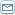# Conmathematics : The Superultramodern Mathematical Science

 By: Dr Kedar JoshiThe term Conmathematics [ invented by Kedar Joshi (b.1979), Cambridge (England), i.e. myself ] means Conceptual Mathematics. It is a meta - mathematical system that defines the structure of Superultramodern Mathematical Science. It has four main components. 1. Conmathematical Definition of Mathematics : a) Conmathematical definition of pure mathematics - Pure mathematics is a system of 100% precise propositions believed to be 99.999...% certain ( the 0.00...1 % margin in the belief being for the sake of universal doubt : the principle that 'anything may be possible.'). Precise means every term of a proposition is absolutely clarified, and every non - axiomatic proposition is supported on the basis of axiomatic one/s leaving no doubt, except the universal doubt. It is very possible that some of the mathematical propositions are not clear ( or understandable ) to some persons. And, some of the propositions are less than 99.999...% certain to some persons. This possibility effectively gives rise to the possibility of multiple mathematical systems according to the nature of the individuals concerned, though truth is believed to be existing independent of individual minds. The principle of universal doubt makes it necessary to review the mathematical systems continuously so that in future they may not be seen to be mathematical at all. b) Conmathematical definition of applied mathematics - Applied mathematics is a system of propositions constructed by applying some or all of the pure mathematical propositions to deal with ( i.e. to explain and / or predict ) phenomena that are believed to be less certain than the phenomena expressed by pure mathematical propositions. For example, I cannot believe the law of gravity ( the law that every matter, in principle, attracts every matter out of some force or curvature of space - time ) to be 99.999...% certain, as I can imagine a universe where the law of gravity is invalid ( i.e. where every matter distracts every matter out of some force or absence of space - time curvature ).2. Philosophy as Mathematics : According to the conmathematical definition of mathematics, the core ideas in ultramodern science / philosophy, though appearing to be philosophical, are, in fact, mathematical. For example, the axiomatic component of the NSTP ( Non - Spatial Thinking Process ) theory is pure mathematical, while its hypothetical component is applied mathematical. 3. Conceptual Reconstruction of Pure Mathematics : It entails some flaws in modern pure mathematics and the ultramodern reconstruction of pure mathematics, free of those flaws. Some of the flaws are mentioned below. a) Flaw in the concept of hyperspace - The Joshian conjecture of 3 dimensional space [ that space, whether appearance or reality, can have 3 and only 3 dimensions ( The conjecture is based on two grounds : i. The NSTP theory implies falsehood of the ontology of general relativity. ii. Four or higher dimensional space cannot justifiably be imagined. ) ] implies that the concept of hyperspace is invalid. And the flaw in the concept of hyperspace has following implications : i. The Riemann hypothesis ( which asserts that all interesting / non - trivial solutions of the zeta function equation lie on a straight line Re (z) = 1 / 2 ) shall never be proved as it is based on the concept of four - dimensional space. ( Then still how the Riemann hypothesis turns out to be correct for the first 1,500,000,000 solutions is in the same category as the mathematical / experimental success of the general relativity, despite of the background physics of the NSTP theory. )ii. The Poincare conjecture [ if 3 dimensional sphere ( the set of points in 4 dimensional space at unit distance from the origin ) is simply connected ] shall neither be proved nor be disproved as it is based on the concept of four - dimensional space as well. iii. Andrew Wiles' proof ( entitled : Modular elliptic curves and Fermat's last theorem ) of Fermat's last theorem ( the theorem that there are no whole number solutions to the equation x^n + y^n = z^n for n greater than 2 ) is flawed as it is also based on the concept of four - dimensional space. b) Flaw in the concept of irrational number - An irrational number ( e.g. Ö 2 ) is not really a number at all as no number can be a square root of 2. 'Ö 2', for example, is a mere symbolic way of saying square root of 2 without actually presenting it, as any way it does not exist.4. Conmathematical Foundations of Pure Mathematics - These are in contrast with the symbolic or, in particular, set theoretic foundations of pure mathematics ( as laid out in Bertrand Russell's Principia Mathematica ). The conmathematical foundations are conceptual ( though symbolism itself is a concept ) which attempt to define number, for example, as a symbolic representation of quantity and justify the equality a + b = b + a on the reason that in scalar addition order is irrelevant ( and, if possible, to decompose this concept or a group of concepts further ).
Science
 • How To Determine If Wind Power Will Work For Your Home • The Hoof in Sciences, Bible and Quran • Science of Diamonds • Cool Science Projects for Kids • Learning About Prehistoric Animals Through Fossil Eggs • The Magnificent Planet We Call Home • Rocket Propulsion Into Space • The Impossible Machine • Does Clean Mean Extreme Green? • Mosquitoes like my blood • The Compton Effect to Electromagnetic Waves • Technology Helps You Learn Basic Spanish • Dna Testing Improves Identification of Survivors • Math Tutor - to Do Away With Mathematics Blues • Drug Delivery System • Windmill Site Selection in Andaman, India : Remote Sensing & GIS • Therapeutic Learning Center - Holisticjunction School • The Incredible History of Venus • Devil in Evolution » More on Most Popular Science Articles